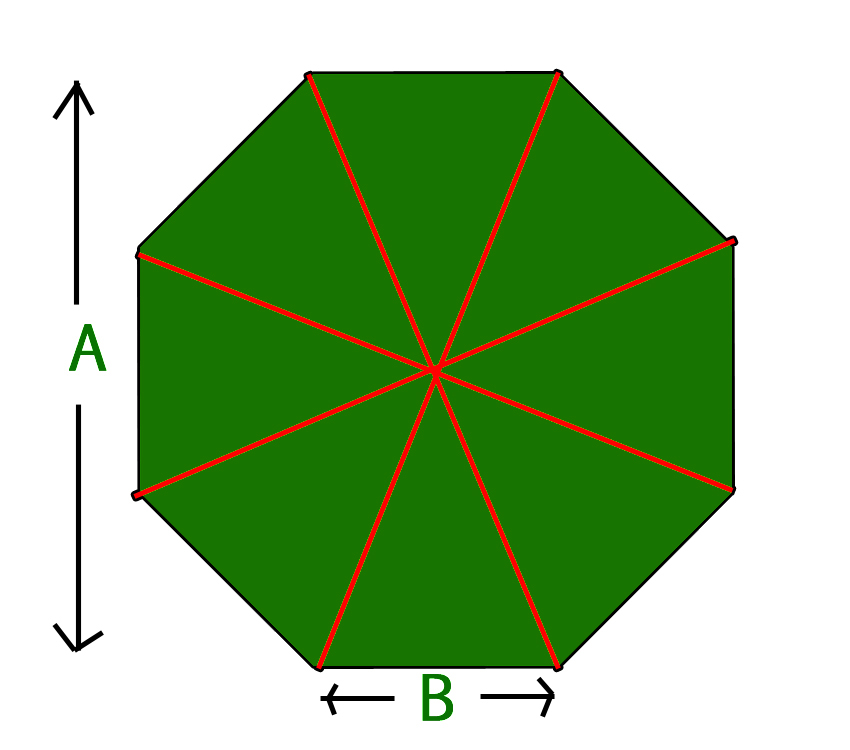# Program to calculate Area Of Octagon

A regular octagon is a closed figure with sides of the same length and internal angles of the same size. It has eight lines of reflective symmetry and rotational symmetry of order 8. The internal angle at each vertex of a regular octagon is 135°. The central angle is 45°.

Properties :

Convex polygon, Equilateral polygon, Isogonal figure, Isotoxal figure, Cyclic.Formula :

`Area : 2 × (side length)² × (1+sqrt(2))`

Examples :

```Input : side = 3
Output : Area of Regular Octagon = 43.4558

Input : side = 4
Output : Area of Regular Octagon = 77.2548
```

## C++

 `// CPP program to find area of octagon ` `#include ` `using` `namespace` `std; ` ` `  `// Utility function ` `double` `areaOctagon(``double` `side) ` `{ ` `    ``return` `(``float``)(2 * (1 + ``sqrt``(2)) *  ` `                   ``side * side); ` `} ` ` `  `// Driver Code ` `int` `main() ` `{ ` `    ``double` `side = 4; ` `    ``cout << ``"Area of Regular Octagon = "` `         ``<< areaOctagon(side) << endl; ` `    ``return` `0; ` `} `

## Java

 `// Java Program to find  ` `// area of Octagon. ` `import` `java.io.*; ` ` `  `class` `GFG ` `{    ` `    ``// utility function ` `    ``static` `double` `areaOctagon(``double` `side) ` `    ``{ ` `    ``return` `(``float``)(``2` `* (``1` `+ Math.sqrt(``2``))  ` `                          ``* side * side); ` `    ``} ` `     `  `    ``// driver code ` `    ``public` `static` `void` `main(String arg[]) ` `    ``{ ` `        ``double` `side = ``4``; ` `        ``System.out.print(``"Area of Regular Octagon = "`   `                          ``+ areaOctagon(side)); ` `    ``} ` `} ` ` `  `// This code is contributed by Anant Agarwal. `

## Python3

 `# Python3 program to  ` `# find area of octagon ` ` `  `import` `math ` ` `  `# Utility function ` `def` `areaOctagon(side): ` `    ``return` `(``2` `*` `(``1` `+` `(math.sqrt(``2``))) ``*` `side ``*` `side) ` ` `  `# Driver function ` `side ``=` `4` `print``(``"Area of Regular Octagon ="``, ` `       ``round``(areaOctagon(side), ``4``)) ` ` `  `# This code is contributed ` `# by Azkia Anam. `

## C#

 `// C# Program to find  ` `// area of Octagon. ` `using` `System; ` ` `  `class` `GFG ` `{  ` `    ``// utility function ` `    ``static` `double` `areaOctagon(``double` `side) ` `    ``{ ` `    ``return` `(``float``)(2 * (1 + Math.Sqrt(2))  ` `                        ``* side * side); ` `    ``} ` `     `  `    ``// Driver code ` `    ``public` `static` `void` `Main() ` `    ``{ ` `        ``double` `side = 4; ` `        ``Console.WriteLine(``"Area of Regular Octagon = "` `                          ``+ areaOctagon(side)); ` `    ``} ` `} ` ` `  `// This code is contributed by vt_m. `

## PHP

 ` `

Output :

```Area of Regular Octagon = 77.2548
```

Attention reader! Don’t stop learning now. Get hold of all the important DSA concepts with the DSA Self Paced Course at a student-friendly price and become industry ready.

My Personal Notes arrow_drop_upCheck out this Author's contributed articles.

If you like GeeksforGeeks and would like to contribute, you can also write an article using contribute.geeksforgeeks.org or mail your article to contribute@geeksforgeeks.org. See your article appearing on the GeeksforGeeks main page and help other Geeks.

Please Improve this article if you find anything incorrect by clicking on the "Improve Article" button below.

Improved By : vt_m